• Last Updated : 26 Jul, 2019

In C#, MaskedTextBox control gives a validation procedure for the user input on the form like date, phone numbers, etc. Or in other words, it is used to provide a mask which differentiates between proper and improper user input. In MaskedTextBox control, you can mask the input of the MaskedTextBox using Mask Property which will be used at runtime to enter some specific type of input in the box.
For example, if you want to enter the phone number in the MaskedTextBox, then you will use the mask property. This property converts the input in the phone number format. It is the default property of MaskedTextBox and if you try to change the mask when the MaskedTextBox already masked with the previous mask. So, it will relocate that input according to the new mask and if it fails in relocation, then it will clear the existing input from the MaskedTextBox. The default value of this property is an empty string, means it can contain any string. You can set this property in two different ways:

1. Design-Time: It is the easiest way to set the Mask property of the MaskedTextBox as shown in the following steps:

• Step 1: Create a windows form as shown in the below image:
Visual Studio -> File -> New -> Project -> WindowsFormApp• Step 2: Next, drag and drop the MaskedTextBox control from the toolbox on the form. As shown in the below image: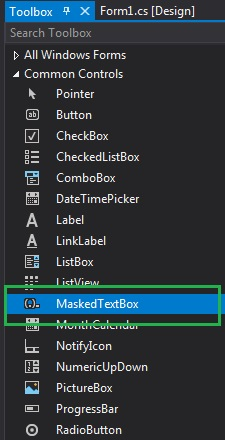• Step 3: After drag and drop you will go to the properties of the MaskedTextBox and set the Mask property of the MaskedTextBox. As shown in the below image: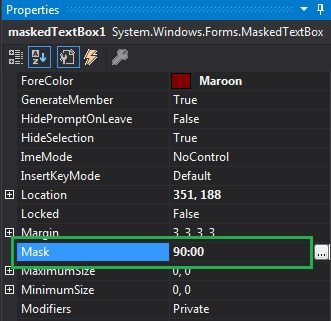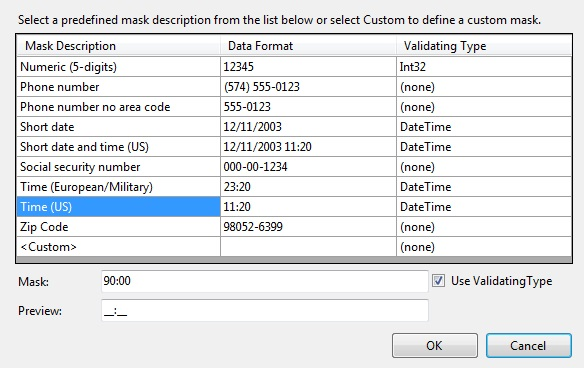Output: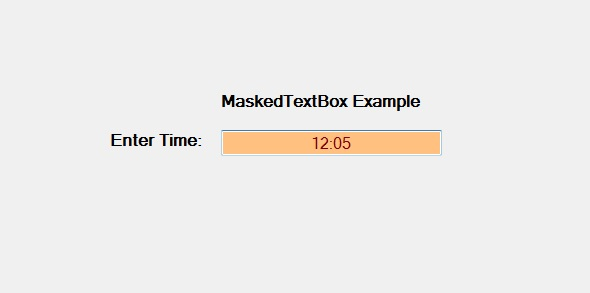2. Run-Time: It is a little bit trickier than the above method. In this method, you can set the Mask property of the MaskedTextBox control programmatically with the help of given syntax:

`public string Mask { get; set; }`

Here, the string indicates the current mask. If the string applied to this property does not belong to a valid mask, then it will throw an InvalidEnumArgumentException. The following steps show how to set the Mask property of the MaskedTextBox dynamically:

```// Creating a MaskedTextBox
```
```// Setting the mask
```
• Step 3: And last add this MaskedTextBox control to the form using the following statement:
```// Adding MaskedTextBox control on the form
```

Example:

 `using` `System;``using` `System.Collections.Generic;``using` `System.ComponentModel;``using` `System.Data;``using` `System.Drawing;``using` `System.Linq;``using` `System.Text;``using` `System.Threading.Tasks;``using` `System.Windows.Forms;`` ` `namespace` `WindowsFormsApp38 {`` ` `public` `partial` `class` `Form1 : Form {`` ` `    ``public` `Form1()``    ``{``        ``InitializeComponent();``    ``}`` ` `    ``private` `void` `Form1_Load(``object` `sender, EventArgs e)``    ``{``        ``// Creating and setting the  ``        ``// properties of the Label``        ``Label l1 = ``new` `Label();``        ``l1.Location = ``new` `Point(413, 98);``        ``l1.Size = ``new` `Size(176, 20);``        ``l1.Text = ``" Example"``;``        ``l1.Font = ``new` `Font(``"Bell MT"``, 12);`` ` `        ``// Adding label on the form``        ``this``.Controls.Add(l1);`` ` `        ``// Creating and setting the ``        ``// properties of the Label``        ``Label l2 = ``new` `Label();``        ``l2.Location = ``new` `Point(242, 135);``        ``l2.Size = ``new` `Size(126, 20);``        ``l2.Text = ``"Phone number:"``;``        ``l2.Font = ``new` `Font(``"Bell MT"``, 12);`` ` `        ``// Adding label on the form``        ``this``.Controls.Add(l2);`` ` `        ``// Creating and setting the ``        ``// properties of MaskedTextBox``        ``MaskedTextBox m = ``new` `MaskedTextBox();``        ``m.Location = ``new` `Point(374, 137);``        ``m.Mask = ``"00/00/0000"``;``        ``m.Size = ``new` `Size(176, 20);``        ``m.Name = ``"MyBox"``;``        ``m.BorderStyle = BorderStyle.Fixed3D;``        ``m.BackColor = Color.LightBlue;``        ``m.ForeColor = Color.HotPink;``        ``m.Font = ``new` `Font(``"Bell MT"``, 18);`` ` `        ``// Adding MaskedTextBox``        ``// control on the form``        ``this``.Controls.Add(m);``    ``}``}``}`

Output: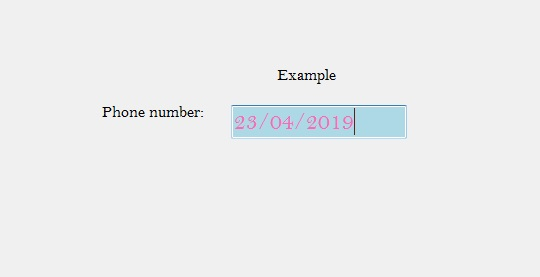My Personal Notes arrow_drop_up